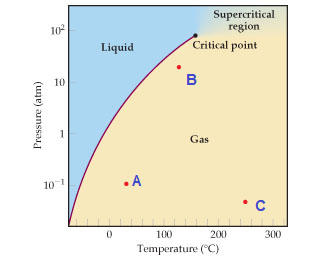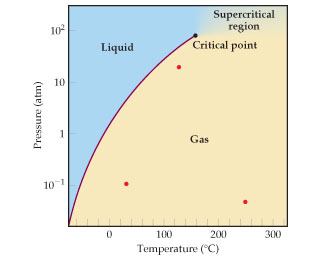# Problem: The phase diagram for SO2 is shown here.At which of the three points marked in red does SO2(g) most closely approach ideal-gas behavior?

###### FREE Expert Solution

In this problem, we are asked which of the three red points would SO2(g) most closely approach ideal-gas behavior.

We can use the phase diagram to answer the given questions

Recall that a phase diagram shows the transition of matter between solid, liquid, and gas phases as temperature and pressure changes.

In a phase diagram, the different phases can be identified by their location:

• Solid: can be found at high pressure and low temperature.
• Liquid: can be found between the solid and gas regions.
• Gas: can be found at low pressure and high temperature.

The given phase diagram is:

• Let us label the three points as A, B, and C.91% (268 ratings)###### Problem Details

The phase diagram for SO2 is shown here.At which of the three points marked in red does SO2(g) most closely approach ideal-gas behavior?